• Hash256
• Hash160
• Reverse Bytes
• Satoshis

# Public Key

A unique number mathematically generated from a private key.

A `public key` is like an account number that you use to receive bitcoins.

It is created from your `private key`, which is like a password for that account number.

#### Try it! - Generate Public Key

Compressed
Uncompressed

You should never enter your private key in to a website, or use a private key generated by a website. Websites can save these private keys and use them to steal any bitcoins you send to its address.

## How do you generate a public key?

You use your `private key` (which is just a big random number) to generate a corresponding public key.

You perform elliptic curve multiplication using your private key, which will give you a final resting point on the elliptic curve. The `x` and `y` coordinate of this point is your `public key`.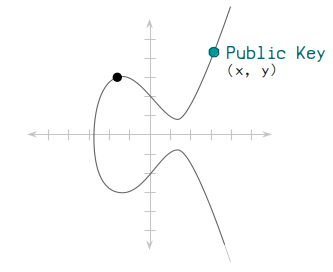### Code

Here’s some basic code for creating a `public key` from a `private key`.

I haven’t explained how the elliptic curve mathematics works, but I’ve included this code anyway to show how you can get started with calculating a `public key` for yourself.

``````# example private key

# --------------------------
# Secp256k1 Curve Parameters
# --------------------------
# y^2 = x^3 + ax + b
\$a = 0
\$b = 7 # using global variables for convenience

# prime modulus
\$p = 2 ** 256 - 2 ** 32 - 2 ** 9 - 2 ** 8 - 2 ** 7 - 2 ** 6 - 2 ** 4 - 1

# number of points on the curve
\$n = 115792089237316195423570985008687907852837564279074904382605163141518161494337

# generator point (the starting point on the curve used for all calculations)
\$g = {
x: 55066263022277343669578718895168534326250603453777594175500187360389116729240,
y: 32670510020758816978083085130507043184471273380659243275938904335757337482424,
}

# --------------------------
# Elliptic Curve Mathematics
# --------------------------
# Modular Inverse - Ruby doesn't have a built-in function for finding modular inverses, so here's one using the extended Euclidean algorithm.
def modinv(a, m = \$p)
a = a % m if a < 0 # make sure a is positive
prevy, y = 0, 1
while a > 1
q = m / a
y, prevy = prevy - q * y, y
a, m = m % a, a
end
return y
end

# Double - Add a point on the curve to itself.
def double(point)
# slope = (3x^2 + a) / 2y
slope = ((3 * point[:x] ** 2) * modinv((2 * point[:y]))) % \$p # using modular inverse to perform "division"

# new x = slope^2 - 2x
x = (slope ** 2 - (2 * point[:x])) % \$p

# new y = slope * (x - new x) * y
y = (slope * (point[:x] - x) - point[:y]) % \$p

# return x, y coordinates of point
return { x: x, y: y }
end

# double if both points are the same
return double(point1) if point1 == point2

# slope = (y1 - y2) / (x1 - x2)
slope = ((point1[:y] - point2[:y]) * modinv(point1[:x] - point2[:x])) % \$p

# new x = slope^2 - x1 - x2
x = (slope ** 2 - point1[:x] - point2[:x]) % \$p

# new y = slope * (x1 - new x) - y1
y = ((slope * (point1[:x] - x)) - point1[:y]) % \$p

# return x, y coordinates of point
return { x: x, y: y }
end

# Multiply - Use the double and add operations to quickly multiply a point by an integer (e.g. a private key).
def multiply(k, point = \$g) # multiply the generator point by default
# create a copy the initial starting point (for use in addition later on)
current = point

# convert integer to binary representation (for use in the double and add algorithm)
binary = k.to_s(2)

# double and add algorithm for fast multiplication
binary.split("").drop(1).each do |char| # ignore first binary character
# 0 = double
current = double(current)

# 1 = double and add
if char == "1"
end
end

# return the final point
return current
end

# -------------------------
# Private Key To Public Key
# -------------------------
# convert private key to an integer
k = private_key.to_i(16)

# multiply generator point by this private key
point = multiply(k, \$g) # this point is the public key

# convert x and y values of this point to hexadecimal
x = point[:x].to_s(16).rjust(64, "0")
y = point[:y].to_s(16).rjust(64, "0")

# uncompressed public key format (not used much these days, just showing how it looks)
public_key_uncompressed = "04" + x + y

# compressed public key format (every x value has a y that could be one of two possible points)
if (point[:y] % 2 == 0)
prefix = "02" # if y is even
else
prefix = "03" # if y is odd
end

public_key_compressed = prefix + x # only uses the full x coordinate

# -------
# Results
# -------
puts public_key_compressed #=> 02b4632d08485ff1df2db55b9dafd23347d1c47a457072a1e87be26896549a8737``````

## Why is the elliptic curve used?

The use of elliptical curve multiplication gives you a mathematical connection from your private key to your public key. It also has two important properties:

### 1. It’s not known how to work backwards to get the private key.

You can go forwards using elliptic curve multiplication, but you cannot do mathematics to go backwards.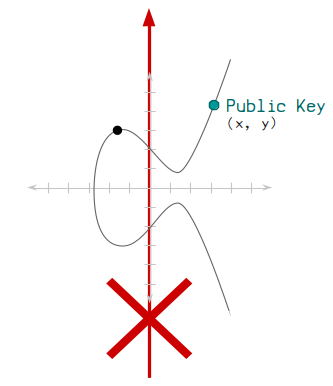This means that there is a mathematical connection going from your private key to your public key, but nobody can use your public key to figure out what your private key is.

Therefore you can give out your public key, but also keep your private key a secret.

### 2. You can prove that you have the private key without giving it away.

Basically, using some more elliptic curve mathematics, you can create a digital signature that proves that you have the corresponding private key for a public key, without ever having to give away your actual private key.

It’s like saying you have the password to an account, but you don’t have to show anyone your actual password to prove it.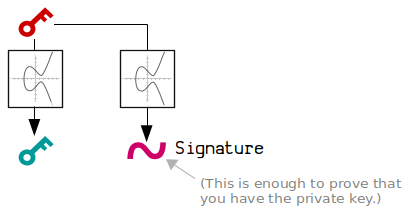This is thanks to the seeming magic of digital signatures, and it’s all made possible through elliptic curve mathematics.

## Public Key Format

A public key is just the `x` and `y` co-ordinate of a point on the elliptic curve. It’s usually stored in hexadecimal format.

There are two formats for public keys:

### 1. Uncompressed

This is the old format. It has generally stopped being used in favor of the shorter compressed format.

Bitcoin originally used both the `x` and `y` coordinate to store the public key.

In this uncompressed format, you just place the `x` and `y` coordinate next to each other, then prefix the whole thing with an `04` to show that it is an uncompressed public key:Here’s an example of what an uncompressed public key looks like:

``public key (uncompressed) = 04b4632d08485ff1df2db55b9dafd23347d1c47a457072a1e87be26896549a87378ec38ff91d43e8c2092ebda601780485263da089465619e0358a5c1be7ac91f4``

### 2. Compressed

However, because the elliptic curve is symmetrical along its x-axis, each `x` coordinate will only ever have one of two possible `y` coordinates.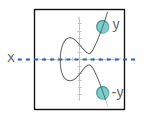And here’s the trick…

• If y is even, it corresponds to one of the points.
• If y is odd, it corresponds to the other.

So in the compressed public key format, we just store the full `x` coordinate, along with a prefix that indicates whether the `y` is even or odd.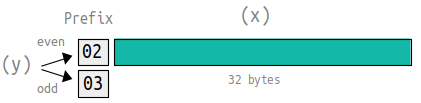We only need to store whether the y coordinate is even or odd.

Here’s an example of what a compressed public key looks like compared to an uncompressed public key:

``````public key (uncompressed) = 04b4632d08485ff1df2db55b9dafd23347d1c47a457072a1e87be26896549a87378ec38ff91d43e8c2092ebda601780485263da089465619e0358a5c1be7ac91f4
public key (compressed)   = 02b4632d08485ff1df2db55b9dafd23347d1c47a457072a1e87be26896549a8737``````

This compressed format ultimately allows us to work out the full `x` and `y` coordinates, but saves a lot of space inside the blockchain (i.e. when we create transactions that lock outputs to specific public keys).

### How to decompress a public key

You can decompress a compressed public key by solving the curve equation `y^2 = x^3 + 7`.

This will give you the missing possible `y` values for the uncompressed key. You can then use the prefix from the compressed key to determine which `y` value to use (because the square root of any number has two possible answers, e.g. `16 = +4 or -4`).

``````# Compressed public key
compressed = "02b4632d08485ff1df2db55b9dafd23347d1c47a457072a1e87be26896549a8737"

# Split compressed key in to prefix and x-coordinate
prefix = compressed[0..1]
x      = compressed[2..-1].to_i(16)

# Secp256k1 curve parameters
p = 0xfffffffffffffffffffffffffffffffffffffffffffffffffffffffefffffc2f

# Work out y values using the curve equation y^2 = x^3 + 7
y_sq = (x**3 + 7) % p # everything is modulo p

# Secp256k1 is chosen in a special way so that the square root of y is y^((p+1)/4)
y = y_sq.pow((p+1)/4, p) # use modular exponentation

# Use prefix to select the correct value for y
# * 02 prefix = y is even
# * 03 prefix = y is odd
if (prefix == "02" && y % 2 != 0) # if prefix is 02 and y isn't even, use other y value
y = (p - y) % p
end
if (prefix == "03" && y % 2 == 0) # if prefix is 03 and y is even, use other y value
y = (p - y) % p
end

# Construct the uncompressed public key
x = x.to_s(16).rjust(64, "0") # convert to hex and make sure it's 32 bytes (64 characters)
y = y.to_s(16).rjust(64, "0")
uncompressed = "04" + x + y

# Result
puts uncompressed #=> 04b4632d08485ff1df2db55b9dafd23347d1c47a457072a1e87be26896549a87378ec38ff91d43e8c2092ebda601780485263da089465619e0358a5c1be7ac91f4``````

## How are public keys used in Bitcoin?

You can give your public key away to people so that they can include it in the the locking script of an output when they create a transaction.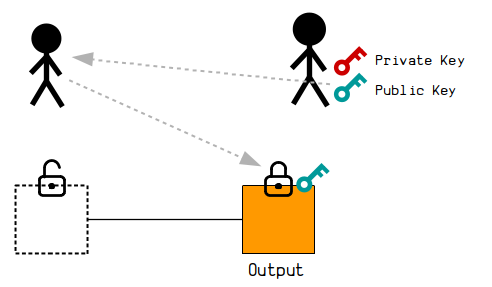We can give people our public key so that they can send us bitcoins. This is called Pay-To-Pubkey (P2PK)

However, in Bitcoin we now more commonly hash160 our public key before giving it away. The public key is then used only when we come to unlocking the output. (The initial lock will then want to check that the public key hashes correctly first before going on to check it against the signature.)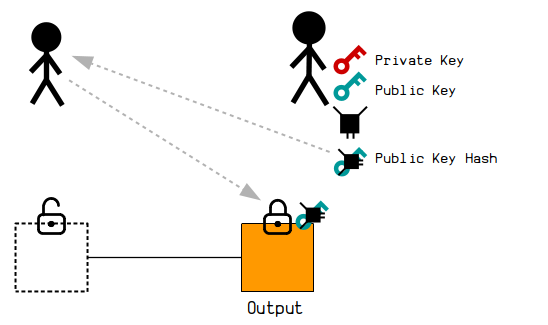The Hash160 of our public key now sits in the lock. This is called Pay-To-PubKey-Hash (P2PKH)

## Where can you find public keys inside the blockchain?

If your looking through raw blockchain data, public keys can typically be found inside transaction data.

In a standard P2PKH transaction, for example:

1. The `public key hash` sits within the locking code (scriptPubKey) of an output.
```01000000017dc9d3eaa91ef9886e48929285243d945a20be621a7483d5442872c2f4bbf432000000004a493046022100ea5812a1cbcf9c8c49fdfb4ed7ef89c05d9d11a5df941fd76546e9031fefbef5022100f5458675ebd56db5517510527afa5ff7c98e08d7ed83a8b180f7ac841531a3ac01ffffffff0100f2052a010000001976a914fc50c5907d86fed474ba5ce8b12a66e0a4c139d888ac00000000
```

Transaction: `24c1f77de7c580eccd46c41d4c708da4ace04880559ee6bce97a67217466d7f7`

Then in the next transaction that spends the bitcoins…

1. The original `public key` can then be found inside the unlocking code (scriptSig) of the input.
```0100000000100000001f7d7667421677ae9bce69e558048e0aca48d704c1dc446cdec80c5e77df7c124000000008b483045022100b92b0d78a1a72b25179260e96a15efe95f98962622fb232f92d6c6ef20e15e9b022061c946c3f976339e370eabd256d91aa4711bb9985330f7d18ee77987b0ca24300141046c04c02f1138f440e8c5e9099db938bfba93d0389528bb7f6bf423ae203a2edcfba133f0409023d7ea13ac01c5aeedaf0bbfbeb8b82e9b48410d93a296da5b0cffffffff0100f2052a010000001976a914e6a874331cddf113e6f424f547aa93c10755d5e688ac00000000
```

Transaction: `33ab606c34dce6e43673d20c1a72c7b0bce314d9d21e227c04092bbdaf8aaed5`

As you can see, the `04` at the start of the public key indicates that it’s an uncompressed public key. This makes it almost twice as long as the compressed public keys typically used today.

## Libraries

In most languages today you can use an existing elliptic curve library to help you create public keys (instead of having to code the mathematics yourself). For example:

``````require 'ecdsa' # Use an elliptic curve library

# This private key is just an example

# Elliptic curve multiplication
group = ECDSA::Group::Secp256k1 # Select the curve used in Bitcoin
point = group.generator.multiply_by_scalar(privatekey.to_i(16)) # Multiply by integer (not hex)

# Convert public key point to a compressed hexadecimal public key
publickey = ECDSA::Format::PointOctetString.encode(point, compression: true).unpack("H*").join

puts publickey``````

### Resources

Hey there, it's Greg.

I'll let you know about cool website updates, or if something seriously interesting happens in bitcoin.

Don't worry, it doesn't happen very often.